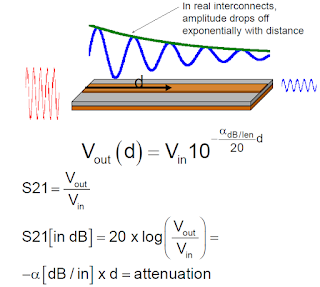## You need to test, we're here to help.

You need to test, we're here to help.

## 11 May 2020

### Reading S-parameters: Monotonic Drop OffsFigure 1. As a signal propagates, the amplitude drops off exponentially with distance due to signal losses.
Last week, we showed how ripple in S-parameters relates to the length of the interconnect. The second common pattern we see when reading plots of S-parameters is the monotonic drop off in the amplitude of the transmission coefficient (S21), commonly referred to as the insertion loss. If we apply a sine wave and look at its amplitude as it moves through that interconnect, we see that it drops off exponentially as shown in Figure 1.

V_Out is shown as V_In times a decaying exponential. (Note that attenuation is usually described using base 10 rather than base e).

Calculating S21 using this expression for V_Out, we find that S21 is simply the attenuation.

So, in the absence of reflections or coupling out, the transmission coefficient is basically the attenuation. We have a direct measure of the attenuation as a function of frequency. Attenuation generally is going to increase with frequency because of dielectric and conductor losses, and S21 will decrease correspondingly.

Let’s look at an example based on an interconnect built on FR4.  First, let’s estimate the expected attenuation due to dielectric loss.

For FR4 the dissipation factor (Df) of about 0.02, dielectric constant (Dk) of about 4, we calculate:

Normalizing for frequency:

This Figure of Merit (FoM) works out to about 0.1dB/inch/GHz, which is useful to remember. So, the attenuation increases with increasing frequency and increasing length.Figure 2. Example measured FR4 interconnect used to confirm the estimated attenuation derived previously. Insertion loss decreases with increasing frequency.
Let’s look at a real measurement of insertion loss for an interconnect. The measurement is shown in Figure 2. The microstrip interconnect based on FR4 was engineered to keep the return loss small, below 12 GHz.

Looking at the measurement we see that the return loss is below 13 dB up to 12 or 13 GHz.  Beyond that frequency there is a rise due to a resonance.  Below that point the interconnect is relatively transparent with a reasonably low reflection coefficient.

We see that monotonic drop-off in the insertion loss.Looking at a frequency of 10 gigahertz,where the drop-off is pretty linear,the drop off in the SDD21,is minus four dB at 10 gigahertz.

Using this along with the 4.5-inch length of the interconnect, we can calculate the FoM which is expected to be about 0.1 dB per inch per gigahertz. The calculation shows an FoM of 0.089, which is very close to the expected 0.1dB/inch/GHz.

In the next post in this series, we'll examine the occurrence of Broad Dips in S-parameters. In the meantime, watch the on-demand webinar Reading S-Parameters Like a Book by Dr. Eric Bogatin, Signal Integrity Evangelist, for a more in-depth treatment of this topic.